## Performance of Mobile Gaming Apps¶

• The Impact of a Business Model on the Relationship between Entrance Timing and Performance among Mobile Games

The goal of this notebook is to guide readers through the process of analyzing Apple AppStore data received from Tilburg University. For more information read through the attached paper that was created for the purpose of finishing the master course Strategy and Business Models.

### 1. Dataset¶

The dataset used in the study is information regarding apps scraped from the Apple AppStore. From those apps their category was collected, average rating, number of ratings, etc. For more details see "Data Preparation".

### 2. Purpose of this study¶

The main purpose of the study is to research the effects of a business model on the performance of Apple AppStore apps. Without going too much into the academic research, the following hypotheses were tested in this paper:

• Hypothesis 1: Both early and late entrants will perform better when they make use of the free revenue model compared to the paid revenue model

• Hypothesis 2: Order of entry and revenue model interact such that free apps will perform better when they are late entrants versus early entrants, whereas paid apps will perform better when they are early entrants versus late entrants.

• Hypothesis 3: Order of entry and technological innovation interact such that apps using technological innovation will perform better when they are early entrants versus late entrants, whereas apps not using technological innovation will perform better when they are late entrants versus early entrants.

I start by cleaning the data and doing some exploratory data analysis (EDA) before doing the statistical analysis. I try to be as clear as possible during the process what is done and for which reason. It should be noted though that for details you might want to look in the paper that was written with my fellow students. Moreover, since I'm not able to share the data this notebook cannot be run on your system. This notebook is to show my thought process when doing the analysis.

### 3. Functions¶

Back to Table of Contents Here are all the functions used in this study. It's a lot, I know! Normally this would all be in a separate .py file, but the goal is to be transparent, so here it is :-)

In :
import re                 as re
import pandas             as pd
import matplotlib.pyplot  as plt
import numpy              as np
import seaborn            as sns

import string

from collections          import Counter
from nltk.corpus          import stopwords
from collections          import Counter

%matplotlib inline

def create_4_timeslots(df):
""" Creates and returns dataframes in 4 timeslots:
- first_seen_2015: Apps in 2015 that were first seen around may
- last_seen_2015: Apps in 2015 that were last seen around september
- first_seen_2017: Apps in 2017 that were first seen around may
- last_seen_2017: Apps in 2017 that were last seen around september
"""
# Create a dataframe with apps in 2015 that were first seen around may
first_seen_2015 = df[(df['timestamp'] == df['firstseen']) & (df['firstseen'].str.contains('2015'))].groupby(by='id').first()
first_seen_2015 = first_seen_2015.reset_index()

# Create a dataframe with apps in 2017 that were first seen around may
first_seen_2017 = df[(df['timestamp'] == df['firstseen']) & (df['firstseen'].str.contains('2017'))].groupby(by='id').first()
first_seen_2017 = first_seen_2017.reset_index()

# Create dataframes with apps in 2015 and 2017 that were last seen around september
last_seen_2015 = df[(df['week'] > 30) & (df['week'] < 40)].groupby(by='id').last().reset_index()
last_seen_2017 = df[df['week'] > 90].groupby(by='id').last().reset_index()

return first_seen_2015, last_seen_2015, first_seen_2017, last_seen_2017

def create_4_equal_timeslots(first_seen_2015, last_seen_2015, first_seen_2017, last_seen_2017):
""" Returns the dataframes of the 4 timeslots so that they include the exact same apps.
- first_seen_2015 and last_seen_2015 contain the same apps
- first_seen_2017 and last_seen_2017 contain the same apps
"""
# Making sure all id's that are in last_seen are also in first_seen
list_ls_2015 = list(last_seen_2015['id'])
first_seen_2015 = first_seen_2015[first_seen_2015['id'].isin(list_ls_2015)]

# Making sure all id's that are in first_seen are also in last_seen
list_fs_2015 = list(first_seen_2015['id'])
last_seen_2015 = last_seen_2015[last_seen_2015['id'].isin(list_fs_2015)]

# Making sure all id's that are in last_seen are also in first_seen
list_ls_2017 = list(last_seen_2017['id'])
first_seen_2017 = first_seen_2017[first_seen_2017['id'].isin(list_ls_2017)]

# Making sure all id's that are in first_seen are also in last_seen
list_fs_2017 = list(first_seen_2017['id'])
last_seen_2017 = last_seen_2017[last_seen_2017['id'].isin(list_fs_2017)]

return first_seen_2015, last_seen_2015, first_seen_2017, last_seen_2017

def create_common_words():
""" Returns a dataframe that contains the most common words for apps found in 2015 and 2017.
I specifically choose to only include first seen apps seeing as that was their initial strategy.
"""
# Create stopwords and add some stopwords that I want removed
stopwords_english = stopwords.words('english')

for word in ['u2022', '', 'u2028', 'will', 'get', 'make', 'like', 'just', 'use', 'u2013', 'let', 'game', '\u2022', '-', '&',
'u', 'e', 'f', 'b', 'c', 'cu', 'bu', 'au', 'fu', 'us', 'go', 'du', 'eu', 'ea', 'uff', 'n', 'one']:
stopwords_english.append(word)

# Create descriptions for each year (first seen), clean it and count the number of words
description = {'2017': '', '2015': ''}

for year, value in description.items():
description[year] = eval('first_seen_{}'.format(year))['description'].str.cat(sep=' ') # create one string of column
description[year] = re.sub('[^a-zA-z]', ' ', description[year]) # only keep letters
description[year] = description[year].replace("\\", "").lower() # remove backslashes and lower the text
description[year] = ' '.join(description[year].split()) # Remove too many spaces
description[year] = description[year].split(' ') # create a list with words
description[year] = Counter(description[year]) # Count how often a word occurs

# Removing stopwords
for word in stopwords_english:
if word in description[year].keys():
del description[year][word]

# Create a dataframe of the count of words for each year for easier readability
df = pd.DataFrame()

for year in ['2015', '2017']:
for action, value in {'word': 0, 'count': 1}.items():
df['{}_{}'.format(year, action)] = [word[value] for word in description[year].most_common(1000)]

return df

def join_first_last(first_seen_2015, last_seen_2015, first_seen_2017, last_seen_2017):
""" Returns the following two dataframes:
- df_2015: An inner join of first_seen_2015 and last_seen_2015
- df_2017: An inner join of first_seen_2015 and last_seen_2017
"""
# Merges first_seen_2015 with last_seen_2015 and adds _first and _last to columns to show which belong to which data
first_seen_2015.columns = [column + "_first" if column != 'id' else 'id' for column in first_seen_2015.columns]
last_seen_2015.columns = [column + "_last" if column != 'id' else 'id' for column in last_seen_2015.columns]
df_2015 = pd.merge(first_seen_2015, last_seen_2015, on='id')

# Merges first_seen_2017 with last_seen_2017 and adds _first and _last to columns to show which belong to which data
first_seen_2017.columns = [column + "_first" if column != 'id' else 'id' for column in first_seen_2017.columns]
last_seen_2017.columns = [column + "_last" if column != 'id' else 'id' for column in last_seen_2017.columns]
df_2017 = pd.merge(first_seen_2017, last_seen_2017, on='id')

return df_2015, df_2017

def get_change(row, column_1, column_2):
""" Used for lambda expression. Compares two columns and gives back a 1 if there's a difference and 0 if there isn't.
"""
if row[column_1] != row[column_2]:
return 1
else:
return 0

def create_change_columns(df_2015, df_2017, columns = ['price', 'screenshots', 'content_rating', 'compatibility',
'size', 'quan_language', 'appversion', 'ratingscurrentversion',
'ratingcurrentversion', 'title']):
""" This will return two dataframes that have a number of new columns that signify the differences between the
value when firstseen and lastseen. For example, the 'price' column might change when first released and seen a
half year later. This function will return dataframes with columns that show whether there was a change (1) or not (0).
"""
for column in columns:
df_2015['change_{}'.format(column)] = df_2015.apply(lambda row: get_change(row, '{}_first'.format(column),
'{}_last'.format(column)), axis = 1)
df_2017['change_{}'.format(column)] = df_2017.apply(lambda row: get_change(row, '{}_first'.format(column),
'{}_last'.format(column)), axis = 1)
return df_2015, df_2017

def show_changes(df_2015, df_2017, columns = ['price', 'screenshots', 'content_rating', 'compatibility', 'size',
'quan_language', 'appversion', 'ratingscurrentversion', 'ratingcurrentversion',
'title']):
""" Prints the number of changes of a column between firstseen and lastseen for 2015 and 2017
"""
for year in ['2015', '2017']:
print('Changes in column between firstseen and lastseen of {}:\n'.format(year))

for column in columns:
changes = eval('df_{}'.format(year))['change_{}'.format(column)].value_counts()

if len(column) < 20:
column = (' '*(20-len(column))) + column
else:
continue

print('{}: \t{} of {}'.format(column, changes, len(eval('df_{}'.format(year)))))
print()

def optimized_for(df_2015, df_2017, devices = ['iphone', 'ipad', 'ipod touch']):
""" Prints how many of the apps are optimized for certain devices based on their release
"""
print('Apps in 2015 that are optimized for the following devices (based on their release): \n')
for value in devices:
df_2015['optimized_for_{}'.format(value)] = df_2015.apply(lambda row: 1 if value in row['compatibility_first'].lower()
else 0, axis = 1)
optimized = df_2015['optimized_for_{}'.format(value)].value_counts()
value = ' '*(20-len(value)) + value
print('{}: \t {} out of {}'.format(value, optimized, len(df_2015)))
print()

print('Apps in 2017 that are optimized for the following devices (based on their release): \n')
for value in devices:
df_2017['optimized_for_{}'.format(value)] = df_2017.apply(lambda row: 1 if value in row['compatibility_first'].lower()
else 0, axis = 1)
optimized = df_2017['optimized_for_{}'.format(value)].value_counts()
value = ' '*(20-len(value)) + value
print('{}: \t {} out of {}'.format(value, optimized, len(df_2017)))
print()

def count_subcategories(df_2015, df_2017):
""" Prints how many apps there are in certain subcategories which are based on certain keywords in an apps description.
"""
slot_games = ['casino', 'slots', 'slot']
driving = ['race', 'drive', 'car', 'driving', 'parking']
puzzle = ['puzzle']
shooter = ['shoot', 'gun', 'pistol', 'sniper', 'war', 'vehicle']

subcategories = {'slot_games': slot_games, 'driving': driving, 'puzzle': puzzle, 'adventure': adventure, 'shooter': shooter}

print('Number of apps in 2015 in the following subcategories:\n')
for category, search_terms in subcategories.items():
amount = len(df_2015[df_2015['description_first'].str.contains('|'.join(search_terms))])

category = " "*(20-len(category)) + category
print(category, ': ', amount, '\tBased on the following terms: {}'.format(', '.join(search_terms)))
print('          Total Apps :  {}'.format(len(df_2015)))

print('\nNumber of apps in 2017 in the following subcategories:\n')
for category, search_terms in subcategories.items():
amount = len(df_2017[df_2017['description_first'].str.contains('|'.join(search_terms))])

category = " "*(20-len(category)) + category
print(category, ': ', amount, '\tBased on the following terms: {}'.format(', '.join(search_terms)))
print('          Total Apps :  {}'.format(len(df_2017)))

def get_difference_rating(row1, row2):
""" Returns the difference in rating between first seen and last seen.
If both first seen and last seen have a rating of -1, then it will return 0
If only first seen has a rating of -1, then it will return the rating of last seen
In all other cases it returns the difference between last seen and first seen
"""
if row1 == -1:
if row1 == row2:
return 0
else:
return row2
else:
return row2 - row1

def return_difference_rating(df_2015, df_2017):
""" Calculates the difference between the rating(s) of an app when it was last seen and when it was first seen.
Returns two dataframes with each two extra column indicating the difference in rating(s).
"""
df_2015['difference_rating'] = df_2015.apply(lambda row: get_difference_rating(row['ratingcurrentversion_first'],
row['ratingcurrentversion_last']), axis = 1)
df_2017['difference_rating'] = df_2017.apply(lambda row: get_difference_rating(row['ratingcurrentversion_first'],
row['ratingcurrentversion_last']), axis = 1)
df_2015['difference_ratings'] = df_2015.apply(lambda row: get_difference_rating(row['ratingscurrentversion_first'],
row['ratingscurrentversion_last']), axis = 1)
df_2017['difference_ratings'] = df_2017.apply(lambda row: get_difference_rating(row['ratingscurrentversion_first'],
row['ratingscurrentversion_last']), axis = 1)
return df_2015, df_2017

def get_revenue_model(row):
""" Returns whether an app if freemium or paid
"""
if row['price_first'] == 0:
return 'Freemium'
else:
return 'Paid'

def get_subcategory(row):
""" Get subcategory based on the amount of keywords are present in the description
"""
slot_games = ['casino', 'slots', 'slot']
driving = ['race', 'drive', 'car', 'driving', 'parking', 'park', 'racing', 'match 3', 'match three', 'match four', 'clues']
puzzle = ['puzzle', 'puzzles', 'puzzling']
shooter = ['shoot', 'gun', 'pistol', 'sniper', 'war', 'vehicle']

subcategories = {'slot_games': slot_games, 'driving': driving, 'puzzle': puzzle, 'adventure': adventure, 'shooter': shooter,
'matching': matching}
count_categories = {'slot_games': 0, 'driving': 0, 'puzzle': 0, 'adventure': 0, 'shooter': 0, 'matching': 0}

description = row['description_first']
description = re.sub('[^a-zA-z]', ' ', description) # only keep letters
description = description.replace("\\", "").lower() # remove backslashes and lower the text
description = ' '.join(description.split()) # Remove too many spaces
description = description.split(' ') # create a list with words
description = Counter(description) # Count how often a word occurs

# Count how many times a certain keyword in one of the categories is seen in a description
for category in subcategories:
for word in subcategories[category]:
if word in description.keys():
count_categories[category] += description[word]

# The category with the most words is returned
if Counter(count_categories).most_common(1) == 0:
return 'Other'
elif Counter(count_categories).most_common(1) == Counter(count_categories).most_common(2):
return 'Other'
else:
return Counter(count_categories).most_common(1)

def create_subcategory(row):
""" Get subcategory based on keywords being present in the description
"""
slot_games = ['casino', 'slots', 'slot']
driving = ['race', 'drive', 'car', 'driving', 'parking', 'park', 'racing']
puzzle = ['puzzle', 'puzzles', 'puzzling', 'match 3', 'match three', 'match four', 'clues']
shooter = ['shoot', 'gun', 'pistol', 'sniper', 'war', 'vehicle']

description = row['description_first']
description = re.sub('[^a-zA-z]', ' ', description) # only keep letters
description = description.replace("\\", "").lower() # remove backslashes and lower the text
description = ' '.join(description.split()) # Remove too many spaces
description = description.split(' ') # create a list with words

for name, category in categories.items():
counter = 0
for word in category:
if (word in description) & (counter == 0):
row[name] = 1
counter += 1
break
else:
continue
if counter == 0:
row[name] = 0
return row

def get_ios_version(row):
return row['compatibility_first'].split('iOS').strip().split(' ').strip().split('.')

def get_content_rating(row):
""" Return the minimum age for a game
"""
rating = row['content_rating_first'].split(" ").replace('+', ' ')
rating = row['content_rating_first'].split('+')

try:
rating = int(rating)
return rating
except:
rating = re.sub('[^0-9]','', row['content_rating_first'])

try:
if int(rating) > 20:
print(row['content_rating_first'])
return int(rating)
except:
'Error'

def create_variables(df_2015, df_2017):
# Create early vs. late mover columns
df_2015['mover'] = 'early'
df_2017['mover'] = 'late'

# Get revenue model
df_2015['revenue'] = df_2015.apply(lambda row: get_revenue_model(row), axis = 1)
df_2017['revenue'] = df_2017.apply(lambda row: get_revenue_model(row), axis = 1)

# Get the subcategory
df_2015 = df_2015.apply(lambda row: create_subcategory(row), axis = 1)
df_2017 = df_2017.apply(lambda row: create_subcategory(row), axis = 1)

# Get optimized for ipod touch
df_2015['optimized_ipod_touch'] = df_2015.apply(lambda row:1 if 'ipod touch' in row['compatibility_first'].lower()
else 0,axis=1)
df_2017['optimized_ipod_touch'] = df_2017.apply(lambda row:1 if 'ipod touch' in row['compatibility_first'].lower()
else 0,axis=1)

# Get the lowest version of iOS for which the app will work
df_2015['ios_version'] = df_2015.apply(lambda row: get_ios_version(row), axis = 1)
df_2017['ios_version'] = df_2017.apply(lambda row: get_ios_version(row), axis = 1)

# content rating
df_2015['content_rating'] = df_2015.apply(lambda row: get_content_rating(row), axis = 1)
df_2017['content_rating'] = df_2017.apply(lambda row: get_content_rating(row), axis = 1)

return df_2015, df_2017

def show_correlation_matrix(df):
sns.set(style="white")
corr = df.corr()

# Generate a mask for the upper triangle

# Set up the matplotlib figure
f, ax = plt.subplots(figsize=(11, 9))

# Generate a custom diverging colormap
cmap = sns.diverging_palette(220, 10, as_cmap=True)

# Draw the heatmap with the mask and correct aspect ratio
square=True, linewidths=.5, cbar_kws={"shrink": .5}, annot=True, fmt=".2f")

def get_innovation(row):
# Technological Innovation (TI)
innovation = ['gyroscope', 'accelerometer', 'vr', 'ar', 'a.r', 'vr-', 'iamcardboard',
'fibrum', 'homido', 'zeiss', 'beenoculus', 'colorcross', 'airvr', 'gyrometer', 'prodji', 'advanceddji',
'prodroneprix', 'onelick', 'vrarchos', 'vrdive', 'vrfreefly', 'gamepad', 'bluetooth']

description = row['description_first']
description = re.sub('[^a-zA-z]', ' ', description) # only keep letters
description = description.replace("\\", " ").lower() # remove backslashes and lower the text
description = ' '.join(description.split()) # Remove too many spaces
description = description.split(' ') # create a list with words

row['innovation'] = 0
for word in innovation:
for word_2 in description:
if word == word_2:
row['innovation'] = 1

description = re.sub('[^a-zA-z]', ' ', row['description_first']).lower().replace('\\', '')
terms = ['augmented reality', 'virtual reality', 'motion control', 'tilt the device', 'tilting the device',
for term in terms:
if term in description:
row['innovation'] = 1
if ('vr-' in row['description_first'].lower().replace('\\', '')):
row['innovation'] = 1
if ('ar-' in row['description_first'].lower().replace('\\', '')):
row['innovation'] = 1

return row


### 4. Data Preparation¶

The dataset app_details contain information regarding all apps in the Apple AppStore in the last 3 years.

In :
df = pd.read_csv('Data set/app_details.csv', low_memory=False)


#### 4.1 Time Slots¶

I want to see the difference in performance between early and late movers after five months of being released. Therefore, four timeslots needs to be created:

• Apps first seen in 2015 (i.e., first_seen_2015)
• Apps 5 months after first seen in 2015 (i.e., last_seen_2015)
• Apps first seen in 2017 (i.e., first_seen_2017)
• Apps 5 months after first seen in 2017 (i.e., last_seen_2017)

Moroever, it is important that the same apps appear in first_seen_2015 and last_seen_2015. The same for 2017. Then, the datasets will be combined into df_2015 and df_2017 where each datasets contains information regarding an app when it was first seen in year 2015/2017 and 5 months laters.

In :
first_seen_2015, last_seen_2015, first_seen_2017, last_seen_2017 = create_4_timeslots(df)
first_seen_2015, last_seen_2015, first_seen_2017, last_seen_2017 = create_4_equal_timeslots(first_seen_2015, last_seen_2015,
first_seen_2017, last_seen_2017)
df_2015, df_2017 = join_first_last(first_seen_2015, last_seen_2015, first_seen_2017, last_seen_2017)

Numer of records in First Seen 2015: 	10547
Numer of records in Last Seen 2017: 	12958
Numer of records in First Seen 2017: 	12958
Numer of records in Last Seen 2015: 	10547


#### 4.2 EDA¶

Although I highly recommend to do extensive exploratory data analysis before going into the modelling, I'm afraid this notebook will simply be too long so I will show only a few key aspects.
First, I check how many apps were optimzed for certain devices based on their release year.

In :
optimized_for(df_2015, df_2017, devices = ['iphone', 'ipad', 'ipod touch'])

Apps in 2015 that are optimized for the following devices (based on their release):

iphone: 	 10283 out of 10547
ipod touch: 	 10272 out of 10547

Apps in 2017 that are optimized for the following devices (based on their release):

iphone: 	 12897 out of 12958
ipod touch: 	 6566 out of 12958



Next, how many apps had changed certain characteristics from when they were first released to 5 months later was visualized.

In :
df_2015, df_2017 = create_change_columns(df_2015, df_2017)
show_changes(df_2015, df_2017)

Changes in column between firstseen and lastseen of 2015:

price: 	376 of 10547
screenshots: 	201 of 10547
content_rating: 	150 of 10547
compatibility: 	10137 of 10547
size: 	1906 of 10547
quan_language: 	252 of 10547
appversion: 	2442 of 10547
title: 	534 of 10547

Changes in column between firstseen and lastseen of 2017:

price: 	276 of 12958
screenshots: 	148 of 12958
content_rating: 	164 of 12958
compatibility: 	6663 of 12958
size: 	1592 of 12958
quan_language: 	116 of 12958
appversion: 	1956 of 12958
title: 	442 of 12958



Finally, I checked for common words in the description of apps in order to get a feeling for which words might represent certain categories. Using those common words, initial categories were constructed within the category "gaming".

In :
common_words = create_common_words()

Out:
2015_word 2015_count 2017_word 2017_count
0 play 9478 play 10419
1 fun 5750 games 7594
2 features 4192 fun 6914
3 free 4084 free 6241
4 time 3596 time 5306
5 games 3119 features 5032
6 new 3108 new 5024
In :
count_subcategories(df_2015, df_2017)

Number of apps in 2015 in the following subcategories:

slot_games :  1110 	Based on the following terms: casino, slots, slot
driving :  1922 	Based on the following terms: race, drive, car, driving, parking
shooter :  1687 	Based on the following terms: shoot, gun, pistol, sniper, war, vehicle
puzzle :  1196 	Based on the following terms: puzzle
Total Apps :  10547

Number of apps in 2017 in the following subcategories:

slot_games :  387 	Based on the following terms: casino, slots, slot
driving :  3552 	Based on the following terms: race, drive, car, driving, parking
shooter :  3295 	Based on the following terms: shoot, gun, pistol, sniper, war, vehicle
puzzle :  1822 	Based on the following terms: puzzle
Total Apps :  12958


#### 4.3 Creating Final Variables¶

It is important to control for several variables in order to get the most accurate results. The following variables are create:

• Innovation - Whether an app was innovative by using for example the gyrometer
• Early vs. Late mover - Whether an app was an early or late mover (binary)
• Revenue model - Either paid or free model
• Subcategory - Which subcategory an app belongs to within the category "gaming"
• Ipod touch - Whether an app was optimized for the Ipod touch
• Ios version
• Content rating - The number of ratings for an app

The resulting columns are shown at the end.

In :
df_2015, df_2017 = create_variables(df_2015, df_2017)
df_2015 = df_2015.apply(lambda row: get_innovation(row), axis = 1)
df_2017 = df_2017.apply(lambda row: get_innovation(row), axis = 1)
final_2015 = df_2015[['mover', 'revenue', 'change_appversion', 'driving', 'adventure', 'puzzle', 'shooter', 'slot_game',
'optimized_ipod_touch', 'ios_version', 'apps_released', 'content_rating', 'screenshots_last',
'quan_description_first', 'size_first', 'quan_language_first', 'quan_moreapps_first', 'innovation',
'ratings_last']]
final_2017 = df_2017[['mover', 'revenue', 'change_appversion', 'driving', 'adventure', 'puzzle', 'shooter', 'slot_game',
'optimized_ipod_touch', 'ios_version', 'apps_released', 'content_rating', 'screenshots_last',
'quan_description_first', 'size_first', 'quan_language_first', 'quan_moreapps_first', 'innovation',
'ratings_last']]
result = final_2015.append(final_2017)

result['bin_target'] = result.apply(lambda row: 0 if row['ratings_last'] == 0 else 1, axis = 1)
result['content_rating'] = result.apply(lambda row: '> ' + str(row['content_rating']), axis = 1)

In :
result.columns

Out:
Index(['mover', 'revenue', 'change_appversion', 'driving', 'adventure',
'puzzle', 'shooter', 'slot_game', 'optimized_ipod_touch', 'ios_version',
'apps_released', 'content_rating', 'screenshots_last',
'quan_description_first', 'size_first', 'quan_language_first',
'quan_moreapps_first', 'innovation', 'ratings_last', 'bin_target'],
dtype='object')

#### 4.4 Correlation Matrix¶

A correlation matrix is shown for most of the variables in the upcoming model to get a feeling for the relationship between the variables.

In :
corr_df = result.copy()
corr_df['mover'] = pd.Categorical(corr_df['mover']).codes
corr_df['revenue'] = pd.Categorical(corr_df['revenue']).codes
show_correlation_matrix(corr_df)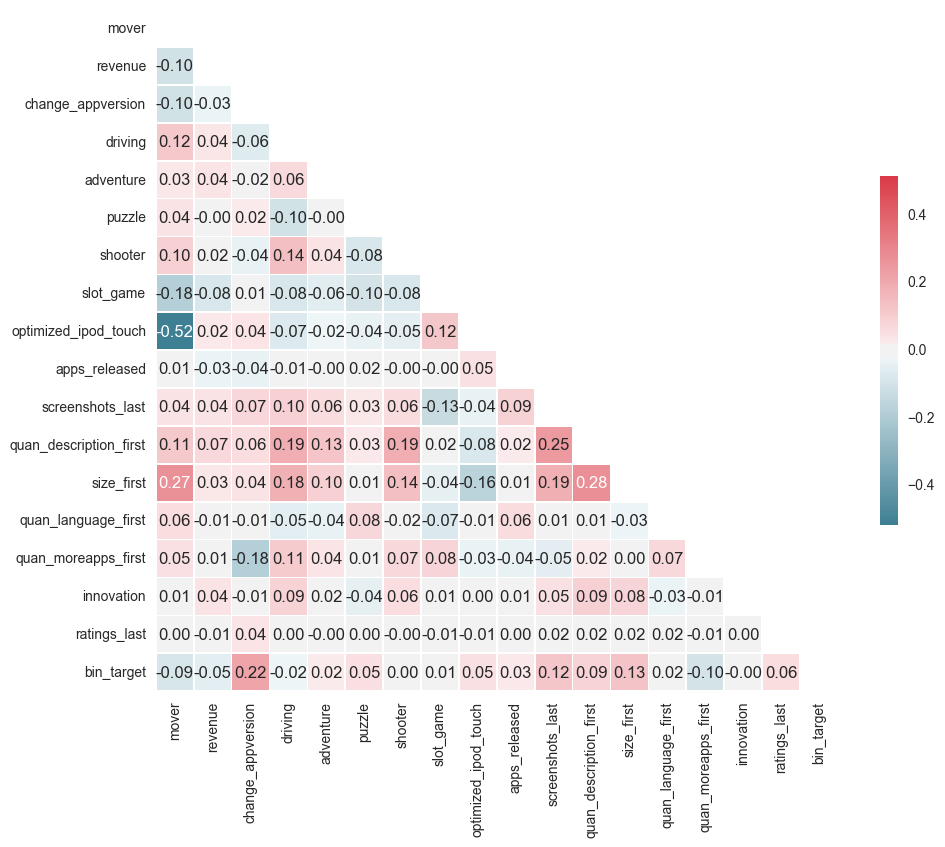### 5 Selecting Statistical Model¶

The target that we are considering is the number of ratings for a particular app. This target can therefore been seen as a count variable.

For count variables there are often two types of models that can be used; a Poisson model or a Negative Binomial model. Let's start with the Poisson model.

The Poisson model assumes equidispersion which means that the conditional mean should be equal to the conditional variance. If the variance is higher than the mean than we can say that the dependent variable is overdispersed (Burger, Van Oort, & Linders, 2009). This is often a problem seeing as in many cases in the real word the variance is much higher than the mean (Atkins, Baldwin, Zheng, Gallop, & Neighbors, 2013). If we take our data set and extract the variance and mean we can clearly see that the ratio of variance to the mean (dispersion parameter) exceeds 1 (equidispersion).

In :
# Checking for dispersion
variance = round(np.var(result['ratings_last']))
mean = int(result['ratings_last'].describe()['mean'])
print('The Poisson model has strict assumptions. One that is often violated is that the mean equals the variance:')
print('Variance: \t\t  {}'.format(variance))
print('Mean:\t\t\t  {}'.format(mean))
print('Dispersion parameter:\t  {}'.format(round(variance/mean)))

The Poisson model has strict assumptions. One that is often violated is that the mean equals the variance:
Variance: 		  6792110
Mean:			  54
Dispersion parameter:	  125780

In :
%%R -i result
model <- glm(ratings_last ~ mover * revenue, data=result, family=quasipoisson)
dispersion <- capture.output({summary(model)})

In :
dispersion = %R dispersion
for line in dispersion[17:-6]:
print(line)

(Dispersion parameter for quasipoisson family taken to be 108013.3)



We can clearly see that the mean doesn't equal the variance and that the dispersion is high. Thus, we need a different model that allows for failing this assumption. The Poisson model can be extended by a more general model which is the negative binomial model. This model allows the mean and varuance to be different. Basically, if the mean and variance were to be the same then Poisson and Negative Binomial would give the same results. However, seeing as that isn't the case, we are going forward with the negative binomial model.

Although we now have chosen the correct model there's still the issue of a high number of zeros. You can see below that the number of zeros in the dataset is 7.56 times the number of non-zeros.

In :
zero = result['ratings_last'][result['ratings_last']==0].count()
not_zero = result['ratings_last'][result['ratings_last']!=0].count()
print('Number of zero ratings: \t\t\t{}'.format(zero))
print('Number of not zero ratings: \t\t\t{}'.format(not_zero))
print('Number of zero ratings compared to non-zero: \t{}'.format(round(float(zero/not_zero), 2)))

Number of zero ratings: 			20758
Number of not zero ratings: 			2747
Number of zero ratings compared to non-zero: 	7.56

In :
# Plot zoomed out
temp = pd.DataFrame(result['ratings_last'].value_counts())
temp = temp.reset_index()

plt.title('Count of the number of ratings')
plt.plot(temp['index'],temp['ratings_last'], 'ro')
plt.show()

# Plot zoomed in
temp = pd.DataFrame(result['ratings_last'][(result['ratings_last'] != 0)].value_counts()).reset_index()
temp = temp[temp['index'] < 100]

plt.title('Count of the number of ratings')
plt.plot(temp['index'], temp['ratings_last'], 'ro')
plt.show()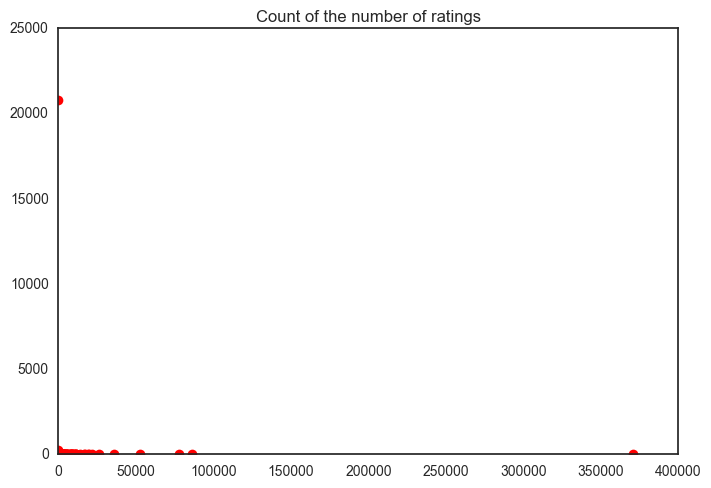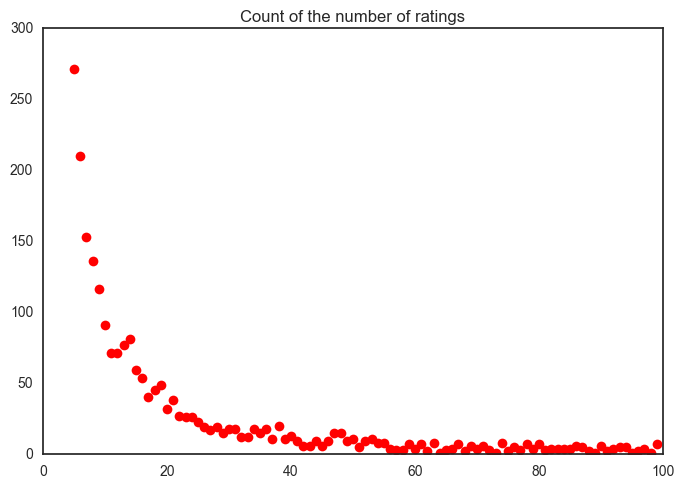Appearantly, we're now dealing with a large inflation of the number of zeros seeing as there is a clear stack of zeros in the data (Atkins et al., 2013). Thus, we need to look at zero-inflated models (Greene, 1994) or hurdle models that account for the number of zeros in the data.

Both hurdle and zero-inflated models account for a large number of zeros, but both do this in a different manner. Hurdle models basically split the data in two parts: zeros and non-zeros. Then a model is used for zero vs. non-zero and a model is used for non-zero values. The interpretability of hurdle models are easier since it generalizes the problem to a zero vs. non-zero logistical model and the remaining non-zeros can be regressed using (I think) a zero-truncated model. Since we don't have repeated observation there is no correlation within individual apps and we don't have to look at mixed models.

Moreover, the zero-inflated binomial regression measures two processes and in our case the zeros might come from the fact that a person downloaded the app and didn't like it enough to leave a rating or that a person didn't downloaded the app and therefore couldn't leave a rating. --> This is debatable and why I prefer to do the Hurdle Model.

#### 6. Statistical Analysis - Revenue Model¶

Dependent Variable:

    - Number of Ratings


Independent Variables:

    - Mover (Early vs. Late)
- Revenue (Paid vs. Freemium)
- Technological Innovation (Yes vs. No)

#### 6.1 Main Effects¶

In :
%%R -i result
library(pscl)
library(MASS)

# Create and execute hurdle model
modelHurdle <- hurdle(formula = ratings_last ~ driving + adventure + puzzle + shooter + slot_game + content_rating +
quan_description_first + size_first + quan_language_first + quan_moreapps_first +
revenue + mover,
dist    = "negbin",
data    = result)

output <- capture.output({summary(modelHurdle)})

In :
# Print results
output = %R output

for line in output[2:-1]:
print(line)

print(' ')

hurdle(formula = ratings_last ~ driving + adventure + puzzle + shooter +
slot_game + content_rating + quan_description_first + size_first +
quan_language_first + quan_moreapps_first + revenue + mover, data = result,
dist = "negbin")

Pearson residuals:
Min        1Q    Median        3Q       Max
-0.47362  -0.14464  -0.11666  -0.09045 184.11382

Count model coefficients (truncated negbin with log link):
Estimate Std. Error z value Pr(>|z|)
(Intercept)             2.963e+00  3.760e-01   7.879 3.29e-15 ***
driving                 3.292e-01  1.895e-01   1.737 0.082301 .
adventure              -3.737e-01  1.589e-01  -2.351 0.018715 *
puzzle                 -3.282e-01  1.441e-01  -2.277 0.022788 *
shooter                -2.936e-01  2.057e-01  -1.427 0.153520
slot_game              -2.807e+00  2.264e-01 -12.398  < 2e-16 ***
content_rating> 17      7.079e-01  3.395e-01   2.085 0.037037 *
content_rating> 4      -8.673e-01  1.861e-01  -4.659 3.17e-06 ***
content_rating> 9      -7.763e-01  2.282e-01  -3.402 0.000668 ***
quan_description_first  1.237e-03  6.859e-05  18.031  < 2e-16 ***
size_first             -4.471e-04  2.749e-04  -1.626 0.103846
quan_language_first     1.322e-01  1.462e-02   9.042  < 2e-16 ***
quan_moreapps_first     2.640e-02  3.005e-02   0.878 0.379680
revenuePaid            -5.046e-01  1.859e-01  -2.715 0.006637 **
moverlate               4.405e-01  1.240e-01   3.553 0.000380 ***
Log(theta)             -3.544e+00  3.351e-01 -10.576  < 2e-16 ***
Zero hurdle model coefficients (binomial with logit link):
Estimate Std. Error z value Pr(>|z|)
(Intercept)            -1.166e+00  8.408e-02 -13.868  < 2e-16 ***
driving                -2.192e-01  7.264e-02  -3.018  0.00254 **
puzzle                  4.775e-01  5.767e-02   8.281  < 2e-16 ***
shooter                 5.568e-02  7.693e-02   0.724  0.46919
slot_game              -2.536e-01  9.611e-02  -2.639  0.00832 **
content_rating> 17     -8.471e-01  1.197e-01  -7.075 1.49e-12 ***
content_rating> 4      -4.767e-01  7.082e-02  -6.731 1.68e-11 ***
content_rating> 9       8.451e-02  9.128e-02   0.926  0.35457
quan_description_first  2.749e-04  3.169e-05   8.674  < 2e-16 ***
size_first              3.335e-03  2.058e-04  16.205  < 2e-16 ***
quan_language_first     1.607e-02  3.385e-03   4.746 2.07e-06 ***
quan_moreapps_first    -1.436e-01  1.100e-02 -13.053  < 2e-16 ***
revenuePaid            -7.992e-01  7.006e-02 -11.408  < 2e-16 ***
moverlate              -8.652e-01  4.692e-02 -18.440  < 2e-16 ***
---
Signif. codes:  0 '***' 0.001 '**' 0.01 '*' 0.05 '.' 0.1 ' ' 1

Theta: count = 0.0289
Number of iterations in BFGS optimization: 87



#### 6.2 Interaction Effects¶

In :
%%R -i result
library(ggplot2)
library(pscl)
library(MASS)
library(boot)
library(pscl)

# Create and execute hurdle model
modelHurdle <- hurdle(formula = ratings_last ~ driving + adventure + puzzle + shooter + slot_game + content_rating +
quan_description_first + size_first + quan_language_first + quan_moreapps_first +
revenue * mover,
dist    = "negbin",
data    = result)

# Comparing with ZINB
zinb <- zeroinfl(ratings_last ~ driving + adventure + puzzle + shooter + slot_game + content_rating +
quan_description_first + size_first + quan_language_first + quan_moreapps_first +
revenue * mover,
data = result, dist = "negbin")
aic <- AIC(modelHurdle, zinb)

output <- capture.output({summary(modelHurdle)})
aic <- capture.output({aic})

In :
aic = %R aic

for line in aic:
print(line)
print('')

            df      AIC
modelHurdle 33 45436.65
zinb        33 45398.22


In :
# Print results
output = %R output

for line in output[2:-1]:
print(line)

print(' ')

hurdle(formula = ratings_last ~ driving + adventure + puzzle + shooter +
slot_game + content_rating + quan_description_first + size_first +
quan_language_first + quan_moreapps_first + revenue * mover, data = result,
dist = "negbin")

Pearson residuals:
Min        1Q    Median        3Q       Max
-0.53139  -0.14540  -0.11745  -0.09123 174.53878

Count model coefficients (truncated negbin with log link):
Estimate Std. Error z value Pr(>|z|)
(Intercept)             2.891e+00  3.521e-01   8.211  < 2e-16 ***
driving                 3.360e-01  1.868e-01   1.799 0.072065 .
adventure              -4.554e-01  1.572e-01  -2.898 0.003758 **
puzzle                 -3.292e-01  1.418e-01  -2.321 0.020301 *
shooter                -2.475e-01  2.038e-01  -1.214 0.224704
slot_game              -2.739e+00  2.216e-01 -12.359  < 2e-16 ***
content_rating> 17      5.706e-01  3.333e-01   1.712 0.086897 .
content_rating> 4      -7.838e-01  1.825e-01  -4.295 1.74e-05 ***
content_rating> 9      -7.609e-01  2.227e-01  -3.417 0.000632 ***
quan_description_first  1.267e-03  6.772e-05  18.708  < 2e-16 ***
size_first             -2.315e-04  2.308e-04  -1.003 0.315810
quan_language_first     1.312e-01  1.455e-02   9.019  < 2e-16 ***
quan_moreapps_first     3.037e-02  2.937e-02   1.034 0.301012
revenuePaid            -1.360e-01  2.167e-01  -0.628 0.530272
moverlate               5.305e-01  1.245e-01   4.262 2.03e-05 ***
revenuePaid:moverlate  -1.895e+00  3.796e-01  -4.992 5.96e-07 ***
Log(theta)             -3.452e+00  3.068e-01 -11.252  < 2e-16 ***
Zero hurdle model coefficients (binomial with logit link):
Estimate Std. Error z value Pr(>|z|)
(Intercept)            -1.175e+00  8.433e-02 -13.929  < 2e-16 ***
driving                -2.179e-01  7.264e-02  -2.999  0.00270 **
puzzle                  4.774e-01  5.766e-02   8.280  < 2e-16 ***
shooter                 5.616e-02  7.694e-02   0.730  0.46541
slot_game              -2.492e-01  9.615e-02  -2.592  0.00954 **
content_rating> 17     -8.483e-01  1.198e-01  -7.084 1.40e-12 ***
content_rating> 4      -4.778e-01  7.084e-02  -6.745 1.53e-11 ***
content_rating> 9       8.121e-02  9.131e-02   0.889  0.37379
quan_description_first  2.743e-04  3.172e-05   8.646  < 2e-16 ***
size_first              3.348e-03  2.062e-04  16.238  < 2e-16 ***
quan_language_first     1.620e-02  3.390e-03   4.779 1.77e-06 ***
quan_moreapps_first    -1.438e-01  1.100e-02 -13.071  < 2e-16 ***
revenuePaid            -7.276e-01  8.344e-02  -8.720  < 2e-16 ***
moverlate              -8.451e-01  4.873e-02 -17.342  < 2e-16 ***
revenuePaid:moverlate  -2.293e-01  1.520e-01  -1.509  0.13131
---
Signif. codes:  0 '***' 0.001 '**' 0.01 '*' 0.05 '.' 0.1 ' ' 1

Theta: count = 0.0317
Number of iterations in BFGS optimization: 66


In :
print('N: {}'.format(len(result[(result['mover']=='late') & (result['innovation'] ==1)])))
print('Mean: {}'.format(result[(result['mover']=='late')& (result['innovation'] ==1)].ratings_last.mean()))
print('Std: {}\n'.format(np.std(result[(result['mover']=='late')& (result['innovation'] ==1)].ratings_last)))

N: 324
Mean: 7.074074074074074
Std: 56.139877725697545



#### 6.3 Truncated Model¶

In :
to_plot = result[result['ratings_last']>0].groupby(by=['mover', 'revenue']).mean().reset_index()
to_plot['revenue_new'] = to_plot.apply(lambda row: 0 if row['revenue'] == 'Paid' else 1, axis = 1)

In :
%%R -i to_plot -w 500 -h 300
library(ggplot2)

ggplot(to_plot, aes(x = revenue_new, y = ratings_last, group = mover, linetype = mover)) + geom_line(size =1) + geom_point(size = 4) +
theme_bw() +
theme(panel.border = element_blank(),
panel.grid.major = element_blank(),
panel.grid.minor = element_blank(),
axis.line = element_line(colour = "black", size = 1, linetype = "solid"),
text = element_text(size=12),
plot.title = element_text(hjust = 0.5)) +
labs(x = 'Revenue Model',
y ="Mean Ratings",
title = "Interaction between Order of Entry on Revenue",
color = 'Order of Entry') +
scale_x_continuous(breaks = round(seq(min(to_plot$revenue_new), max(to_plot$revenue_new), by = 1),1),
expand = c(0.2, 0.2), labels = c('Paid', 'Free'))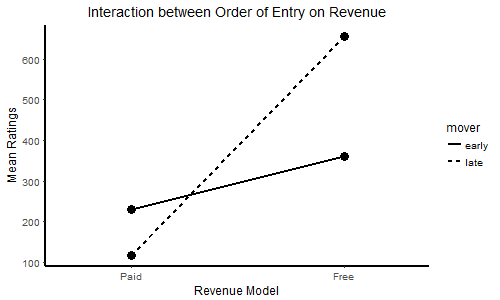#### 6.4 Logistical Model¶

In :
to_plot = result.groupby(by=['mover', 'revenue']).mean().reset_index()
to_plot['revenue_new'] = to_plot.apply(lambda row: 0 if row['revenue'] == 'Paid' else 1, axis = 1)

In :
%%R -i to_plot -w 500 -h 300
library(ggplot2)

ggplot(to_plot, aes(x = revenue_new, y = bin_target ,group = mover, linetype = mover)) + geom_line(size =1) + geom_point(size = 4) +
theme_bw() +
theme(panel.border = element_blank(),
panel.grid.major = element_blank(),
panel.grid.minor = element_blank(),
axis.line = element_line(colour = "black", size = 1, linetype = "solid"),
text = element_text(size=12),
plot.title = element_text(hjust = 0.5)) +
labs(x = 'Revenue Model',
y ="Chance of Rating",
title = "Chance of leaving a Rating between Order of Entry and Revenue",
color = 'Order of Entry') +
scale_x_continuous(breaks = round(seq(min(to_plot$revenue_new), max(to_plot$revenue_new), by = 1),1),
expand = c(0.2, 0.2), labels = c('Paid', 'Free'))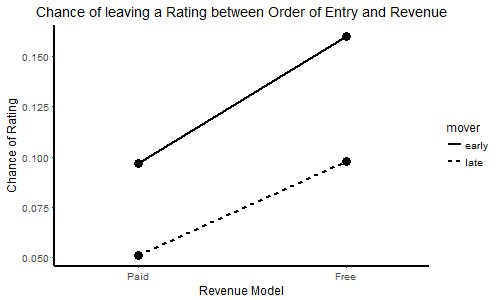#### 6.5 Results¶

Seeing as there are 2 models used in the hurdle model we interpret the results seperately.

Logistical model
The chance of an user leaving a rating for late movers is significantly lower than that for early movers.
In other words, users of late apps are 0.57 times less likely to leave a rating compared to early movers.

The chance of an user leaving a rating for paid apps is significantly lower than that of users of free apps.
In other words, users of paid apps are 0.56 times less likely to leave a rating compared to free apps.

These was no interaction effect of revenue on mover.

Truncated negative binomial model
Later movers have significantly more ratings than early movers.
Free apps get significantly more ratings than paid apps.

Late movers have a higher number of ratings than early movers when it concerns free apps.
This is reversed for paid apps, late movers get lower number of ratings than early movers.
Thus, late movers have a higher number of ratings than early movers. This effect is reversed if it concerns paid apps and is strengthened if it concerns free apps.

### 7. Statistical Analysis - Technological Innovation¶

#### 7.1 Main Effects¶

In :
%%R -i result
library(pscl)

# Create and execute hurdle model
modelHurdle <- hurdle(formula = ratings_last ~ driving + adventure + puzzle + shooter + slot_game + content_rating +
quan_description_first + size_first + quan_language_first + quan_moreapps_first +
innovation + mover,
dist    = "negbin",
data    = result)

# Export output
output <- capture.output({summary(modelHurdle)})

In :
# Print results
output = %R output

for line in output[2:-1]:
print(line)

print(' ')

hurdle(formula = ratings_last ~ driving + adventure + puzzle + shooter +
slot_game + content_rating + quan_description_first + size_first +
quan_language_first + quan_moreapps_first + innovation + mover, data = result,
dist = "negbin")

Pearson residuals:
Min        1Q    Median        3Q       Max
-0.45575  -0.14605  -0.12021  -0.09072 182.22818

Count model coefficients (truncated negbin with log link):
Estimate Std. Error z value Pr(>|z|)
(Intercept)             2.828e+00  3.879e-01   7.289 3.13e-13 ***
driving                 3.237e-01  1.896e-01   1.707 0.087748 .
adventure              -4.380e-01  1.562e-01  -2.804 0.005055 **
puzzle                 -3.120e-01  1.450e-01  -2.152 0.031362 *
shooter                -2.758e-01  2.207e-01  -1.250 0.211404
slot_game              -2.744e+00  2.307e-01 -11.894  < 2e-16 ***
content_rating> 17      6.439e-01  3.507e-01   1.836 0.066307 .
content_rating> 4      -8.208e-01  1.935e-01  -4.241 2.22e-05 ***
content_rating> 9      -8.058e-01  2.357e-01  -3.419 0.000629 ***
quan_description_first  1.239e-03  6.898e-05  17.959  < 2e-16 ***
size_first             -4.578e-04  3.178e-04  -1.441 0.149708
quan_language_first     1.339e-01  1.459e-02   9.180  < 2e-16 ***
quan_moreapps_first     2.638e-02  3.013e-02   0.876 0.381296
innovation              1.534e-01  4.270e-01   0.359 0.719508
moverlate               4.848e-01  1.222e-01   3.969 7.22e-05 ***
Log(theta)             -3.590e+00  3.500e-01 -10.258  < 2e-16 ***
Zero hurdle model coefficients (binomial with logit link):
Estimate Std. Error z value Pr(>|z|)
(Intercept)            -1.2691755  0.0833304 -15.231  < 2e-16 ***
driving                -0.2178318  0.0723816  -3.009  0.00262 **
puzzle                  0.4684511  0.0574275   8.157 3.43e-16 ***
shooter                 0.0643777  0.0766466   0.840  0.40095
slot_game              -0.1466874  0.0952278  -1.540  0.12347
content_rating> 17     -0.8343618  0.1190163  -7.010 2.37e-12 ***
content_rating> 4      -0.4701231  0.0703561  -6.682 2.36e-11 ***
content_rating> 9       0.0586664  0.0906547   0.647  0.51754
quan_description_first  0.0002562  0.0000314   8.161 3.33e-16 ***
size_first              0.0031877  0.0002035  15.667  < 2e-16 ***
quan_language_first     0.0165873  0.0033576   4.940 7.80e-07 ***
quan_moreapps_first    -0.1477630  0.0109449 -13.501  < 2e-16 ***
innovation             -0.2483321  0.1421415  -1.747  0.08062 .
moverlate              -0.7842620  0.0461380 -16.998  < 2e-16 ***
---
Signif. codes:  0 '***' 0.001 '**' 0.01 '*' 0.05 '.' 0.1 ' ' 1

Theta: count = 0.0276
Number of iterations in BFGS optimization: 55



#### 7.2 Interaction Effects¶

In :
%%R -i result
library(ggplot2)
library(pscl)
library(MASS)
library(boot)
library(pscl)

# Create and execute hurdle model
modelHurdle <- hurdle(formula = ratings_last ~ driving + adventure + puzzle + shooter + slot_game + content_rating +
quan_description_first + size_first + quan_language_first + quan_moreapps_first +
innovation * mover,
dist    = "negbin",
data    = result)

# Comparing with ZINB
zinb <- zeroinfl(ratings_last ~ driving + adventure + puzzle + shooter + slot_game + content_rating +
quan_description_first + size_first + quan_language_first + quan_moreapps_first +
innovation * mover,
data = result, dist = "negbin")
aic <- AIC(modelHurdle, zinb)

# Export output
output <- capture.output({summary(modelHurdle)})
aic <- capture.output({aic})

In :
aic = %R aic

for line in aic:
print(line)
print('')

            df      AIC
modelHurdle 33 45599.24
zinb        33 45577.37


In :
# Print results
output = %R output
expCoef = %R expCoef

for line in output[2:-1]:
print(line)

print(' ')

hurdle(formula = ratings_last ~ driving + adventure + puzzle + shooter +
slot_game + content_rating + quan_description_first + size_first +
quan_language_first + quan_moreapps_first + innovation * mover, data = result,
dist = "negbin")

Pearson residuals:
Min       1Q   Median       3Q      Max
-0.4467  -0.1469  -0.1205  -0.0912 180.3122

Count model coefficients (truncated negbin with log link):
Estimate Std. Error z value Pr(>|z|)
(Intercept)             2.849e+00  3.693e-01   7.712 1.24e-14 ***
driving                 3.360e-01  1.889e-01   1.779 0.075291 .
adventure              -4.176e-01  1.561e-01  -2.674 0.007494 **
puzzle                 -3.103e-01  1.449e-01  -2.141 0.032255 *
shooter                -3.554e-01  2.114e-01  -1.681 0.092792 .
slot_game              -2.697e+00  2.279e-01 -11.833  < 2e-16 ***
content_rating> 17      6.763e-01  3.484e-01   1.941 0.052272 .
content_rating> 4      -7.561e-01  1.890e-01  -4.000 6.34e-05 ***
content_rating> 9      -7.210e-01  2.315e-01  -3.115 0.001839 **
quan_description_first  1.217e-03  6.822e-05  17.847  < 2e-16 ***
size_first             -3.605e-04  3.358e-04  -1.074 0.282945
quan_language_first     1.326e-01  1.452e-02   9.136  < 2e-16 ***
quan_moreapps_first     2.098e-02  3.004e-02   0.698 0.484889
innovation              7.041e-01  5.058e-01   1.392 0.163921
moverlate               5.051e-01  1.221e-01   4.137 3.52e-05 ***
innovation:moverlate   -2.644e+00  7.525e-01  -3.514 0.000442 ***
Log(theta)             -3.527e+00  3.301e-01 -10.686  < 2e-16 ***
Zero hurdle model coefficients (binomial with logit link):
Estimate Std. Error z value Pr(>|z|)
(Intercept)            -1.278e+00  8.349e-02 -15.311  < 2e-16 ***
driving                -2.150e-01  7.237e-02  -2.970  0.00298 **
puzzle                  4.664e-01  5.743e-02   8.121 4.62e-16 ***
shooter                 6.050e-02  7.672e-02   0.789  0.43034
slot_game              -1.529e-01  9.529e-02  -1.604  0.10869
content_rating> 17     -8.315e-01  1.191e-01  -6.982 2.91e-12 ***
content_rating> 4      -4.698e-01  7.041e-02  -6.672 2.52e-11 ***
content_rating> 9       5.915e-02  9.071e-02   0.652  0.51438
quan_description_first  2.574e-04  3.142e-05   8.191 2.60e-16 ***
size_first              3.206e-03  2.039e-04  15.719  < 2e-16 ***
quan_language_first     1.655e-02  3.357e-03   4.929 8.26e-07 ***
quan_moreapps_first    -1.476e-01  1.095e-02 -13.486  < 2e-16 ***
innovation              6.614e-02  1.791e-01   0.369  0.71188
moverlate              -7.701e-01  4.645e-02 -16.581  < 2e-16 ***
innovation:moverlate   -7.443e-01  2.960e-01  -2.514  0.01193 *
---
Signif. codes:  0 '***' 0.001 '**' 0.01 '*' 0.05 '.' 0.1 ' ' 1

Theta: count = 0.0294
Number of iterations in BFGS optimization: 58


In :
print('Mean Early entrants + TI: {}'.format(result[(result['mover']=='early') & (result['innovation']==1)].ratings_last.mean()))
print('Std Early entrants + TI: {}\n'.format(np.std(result[(result['mover']=='early') & (result['innovation']==1)].ratings_last)))

print('Mean Late entrants + TI: {}'.format(result[(result['mover']=='late') & (result['innovation']==1)].ratings_last.mean()))
print('Std Late entrants + TI: {}\n'.format(np.std(result[(result['mover']=='late') & (result['innovation']==1)].ratings_last)))

Mean Early entrants + TI: 142.2468085106383
Std Early entrants + TI: 1693.3583321947046

Mean Late entrants + TI: 7.074074074074074
Std Late entrants + TI: 56.139877725697545


In :
print('Mean Early entrants + No TI: {}'.format(result[(result['mover']=='early') & (result['innovation']==0)].ratings_last.mean()))
print('Std Early entrants + No TI: {}\n'.format(np.std(result[(result['mover']=='early') & (result['innovation']==0)].ratings_last)))

print('Mean Late entrants + No TI: {}'.format(result[(result['mover']=='late') & (result['innovation']==0)].ratings_last.mean()))
print('Std Late entrants + No TI: {}\n'.format(np.std(result[(result['mover']=='late') & (result['innovation']==0)].ratings_last)))

Mean Early entrants + No TI: 48.52133436772692
Std Early entrants + No TI: 1323.35594862746

Mean Late entrants + No TI: 58.20294443564983
Std Late entrants + No TI: 3339.6638124301403



#### 7.3 Truncated Model¶

In :
to_plot = result[result['ratings_last']>0].groupby(by=['mover', 'innovation']).mean().reset_index()

In :
%%R -i to_plot -w 500 -h 300
library(ggplot2)

ggplot(to_plot, aes(x = innovation, y = ratings_last ,group = mover, linetype = mover)) + geom_line(size =1) + geom_point(size = 4) +
theme_bw() +
theme(panel.border = element_blank(),
panel.grid.major = element_blank(),
panel.grid.minor = element_blank(),
axis.line = element_line(colour = "black", size = 1, linetype = "solid"),
text = element_text(size=12),
plot.title = element_text(hjust = 0.5)) +
labs(x = 'Technological Innovation',
y ="Mean Ratings",
title = "Interaction between Technological Innovation and Order of Entry",
color = 'Order of Entry') +
scale_x_continuous(breaks = round(seq(min(to_plot$innovation), max(to_plot$innovation), by = 1),1),
expand = c(0.2, 0.2), labels = c('No', 'Yes'))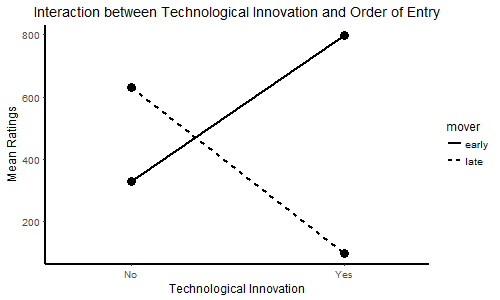#### 7.4 Logistical Model¶

In :
to_plot = result.groupby(by=['mover', 'innovation']).mean().reset_index()

In :
%%R -i to_plot -w 500 -h 300
library(ggplot2)

ggplot(to_plot, aes(x = innovation, y = bin_target ,group = mover , linetype = mover)) + geom_line(size =1) + geom_point(size = 4) +
theme_bw() +
theme(panel.border = element_blank(),
panel.grid.major = element_blank(),
panel.grid.minor = element_blank(),
axis.line = element_line(colour = "black", size = 1, linetype = "solid"),
text = element_text(size=12),
plot.title = element_text(hjust = 0.5)) +
labs(x = 'Technological Innovation',
y ="Chance of Rating",
title = "Chances of leaving a Rating between Order of Entry and TI",
color = 'Order of Entry') +
scale_x_continuous(breaks = round(seq(min(to_plot$innovation), max(to_plot$innovation), by = 1),1),
expand = c(0.2, 0.2), labels = c('No', 'Yes'))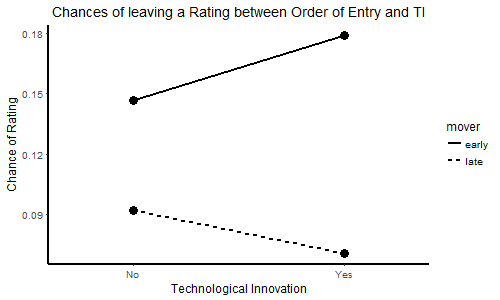#### 7.5 Results¶

Seeing as there are 2 models used in the hurdle model we interpret the results seperately.

Logistical model
The logistical model showed that the chance of an user leaving a rating for late entrants is significantly lower than that for early entrants (p < .001).

Moreover, there was no significant difference in the chance of getting ratings between apps using technological innovation and not using technological innovation (p = .081).

There was an interaction effect of technological innovation on order of entry (p = .131, R2 = .05).

Truncated negative binomial model
The truncated negative binomial model showed that later entrants have on average significantly more ratings than early entrants (p < .001).

Moreover, there was no significant difference between the number of ratings for apps making use of technological innovation and apps not making use of technological innovation (p = .720).

Finally, an interaction was found between technological innovation and order of entry. On average the number of ratings for early entrants making use of technological innovation are higher than the number of ratings for later entrants making use of technological innovation, whereas the number of ratings for later entrants not making use of technological innovation were on average higher than the number of ratings for early entrants not making use of technological innovation (p < .001, R2 = .33).